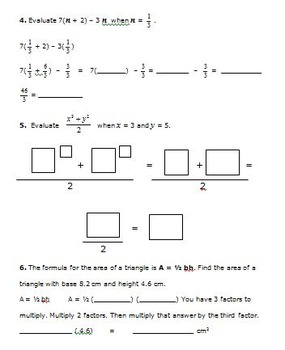# 6th Grade Math - Expressions and Equations Bundle - CCSS 6.EE.1,2,3,4,5,6,7,8,96th
Subjects
Standards
Resource Type
Formats Included
• Zip

### Description

This bundle contains resources covering each common core standard in the 6th grade Expressions and Equations domain.

Click on a link below to view each individual resource description and preview.

Common Core Math 6th Grade - Exponents - CCSS 6.EE.1

Common Core Math 6th Grade Expressions (6.EE.2a,b,c) with I Have-Who Has Game

Common Core Math 6th Grade Equivalent Expressions (6.EE.3,4) with I Have-Who Has

Common Core Math 6th Grade Equations/Inequalities Write Expressions (6.EE.5.6)

Common Core Math 6th Grade - Solve Equations - Graph Inequalities (6.EE.7,8)

Common Core Math Activities 6th Grade (6.EE.9) Tables, Graphs, Equations

The total cost of buying these resources individually is \$28.00.

Thanks for viewing!

Created by Hilda Ratliff
Total Pages
Included
Teaching Duration
N/A
Report this Resource to TpT
Reported resources will be reviewed by our team. Report this resource to let us know if this resource violates TpT’s content guidelines.

### Standards

to see state-specific standards (only available in the US).
Write and evaluate numerical expressions involving whole-number exponents.
Write, read, and evaluate expressions in which letters stand for numbers.
Apply the properties of operations to generate equivalent expressions. For example, apply the distributive property to the expression 3 (2 + 𝘹) to produce the equivalent expression 6 + 3𝘹; apply the distributive property to the expression 24𝘹 + 18𝘺 to produce the equivalent expression 6 (4𝘹 + 3𝘺); apply properties of operations to 𝘺 + 𝘺 + 𝘺 to produce the equivalent expression 3𝘺.
Identify when two expressions are equivalent (i.e., when the two expressions name the same number regardless of which value is substituted into them). For example, the expressions 𝘺 + 𝘺 + 𝘺 and 3𝘺 are equivalent because they name the same number regardless of which number 𝘺 stands for.
Understand solving an equation or inequality as a process of answering a question: which values from a specified set, if any, make the equation or inequality true? Use substitution to determine whether a given number in a specified set makes an equation or inequality true.